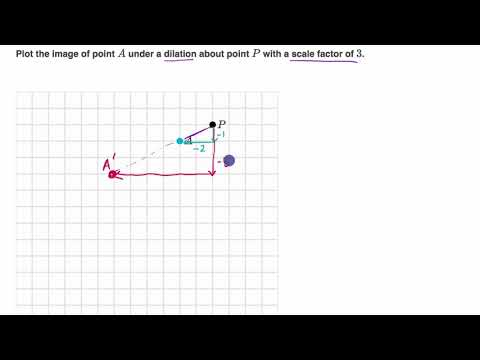Video

# Dilating shapes: expanding (Full video)

Description: Sal is given a rectangle on the coordinate plane and he draws the image of the rectangle under a dilation with scale factor 1 2/3 about an arbitrary point. Created by Sal Khan. Draw the image of ABCP under a dilation whose center is at P and a scale factor is 1 and 2/3. So if we're centering a dilation at P and its scale factor is 1 and 2/3, that means once we perform the dilation, every point is going to be 1 and 2/3 times as far away from P. Well P is 0 away from P, so its image is still going to be at P. Now point C is going to be 1 and 2/3 times as far as it is right now. So let's see, right now it is 6 away.

### Other videos you might be interested in### Dilating points (Full video)